# Linear Discriminant Analysis: how to extract the equation

Member Posts: 10Contributor I
I have a question. LDA analysis is aimed at determining parameters of the hyperplane(s) which are the best separators of observations. If we substitute the real data points into these equations, we can tell whether these points lie above the plane or below the plane and we can do classification.
No matter how much I try and read about Rapidminer I cannot find any explanation how to extract the LDA equation. To make sure that I am understood I uploaded pictures of how this equation is calculated. In the example I took from the internet, the equation I am talking about is function f.
It has to be calculated because otherwise the model could not do classification. However, if I watch the model as the output I only receive a'priori probabilities, not the LDA equation.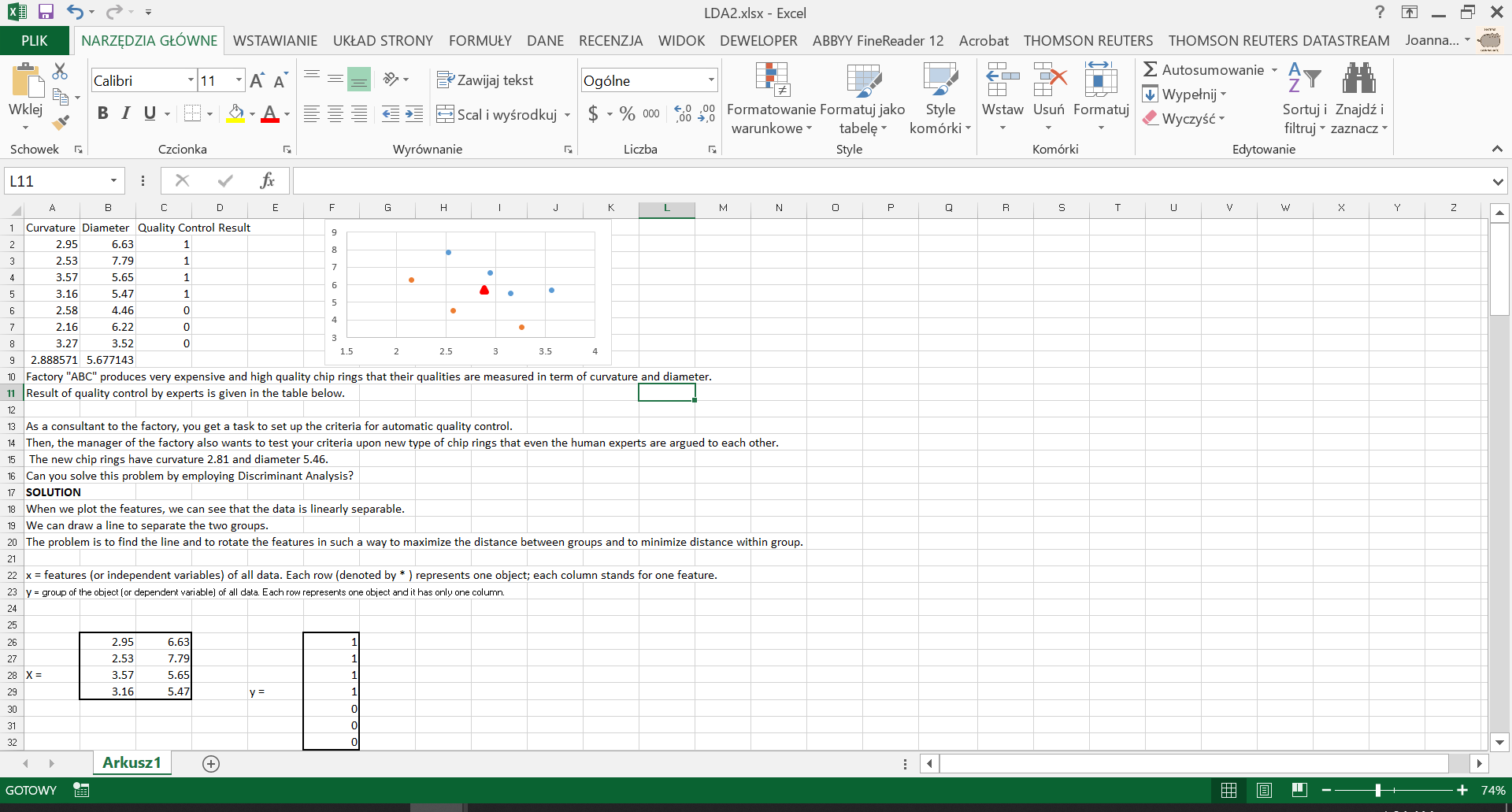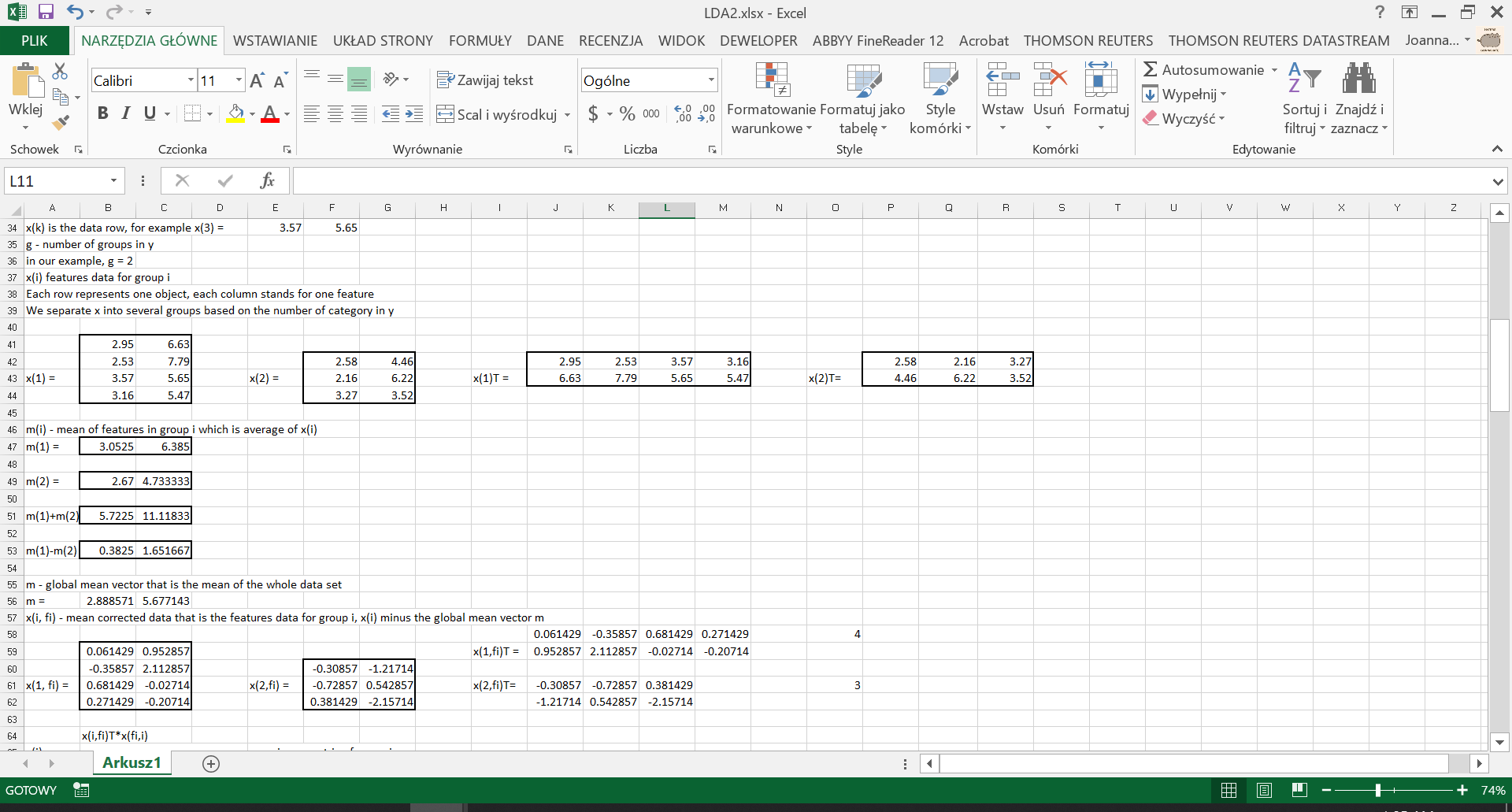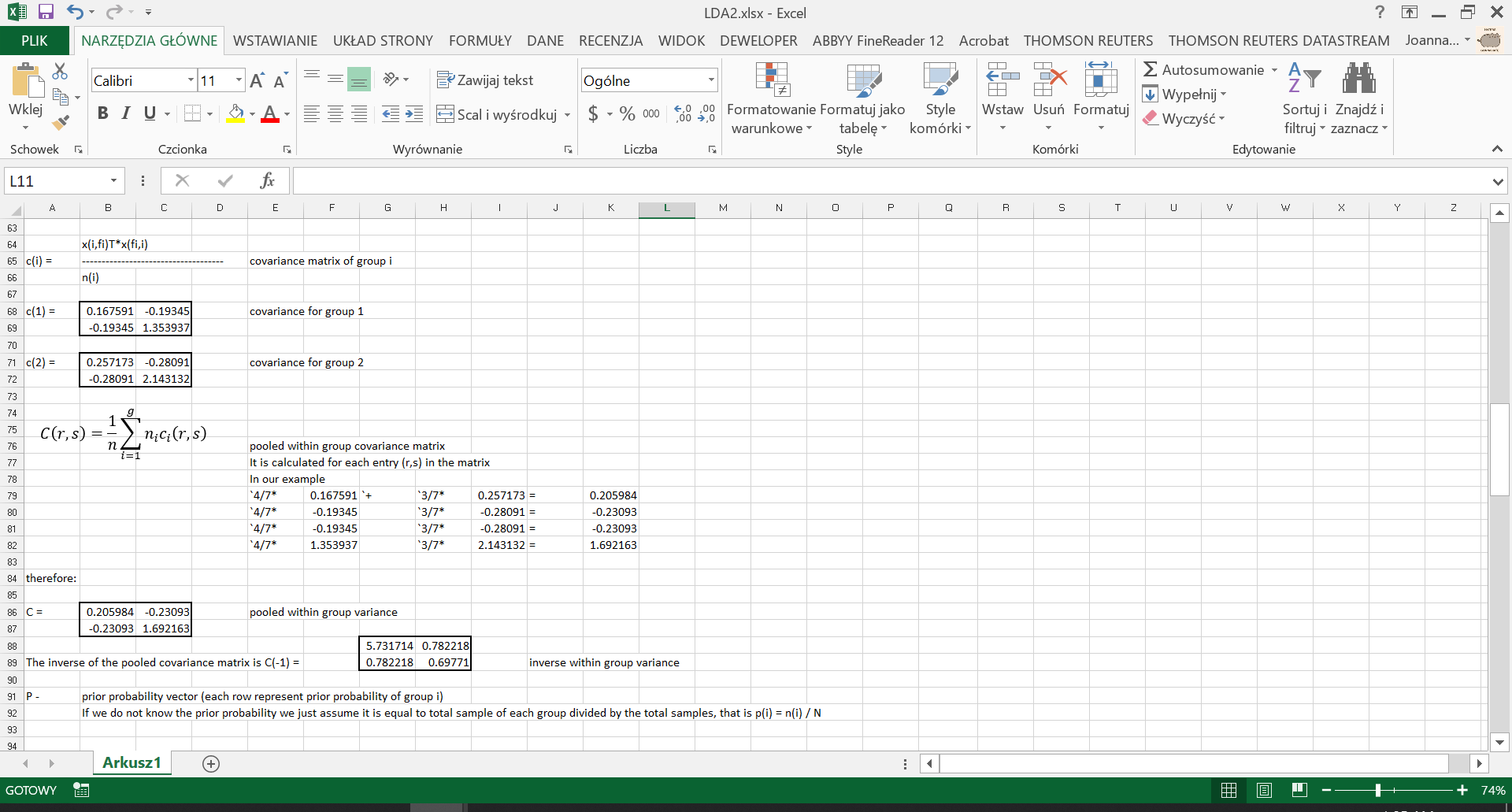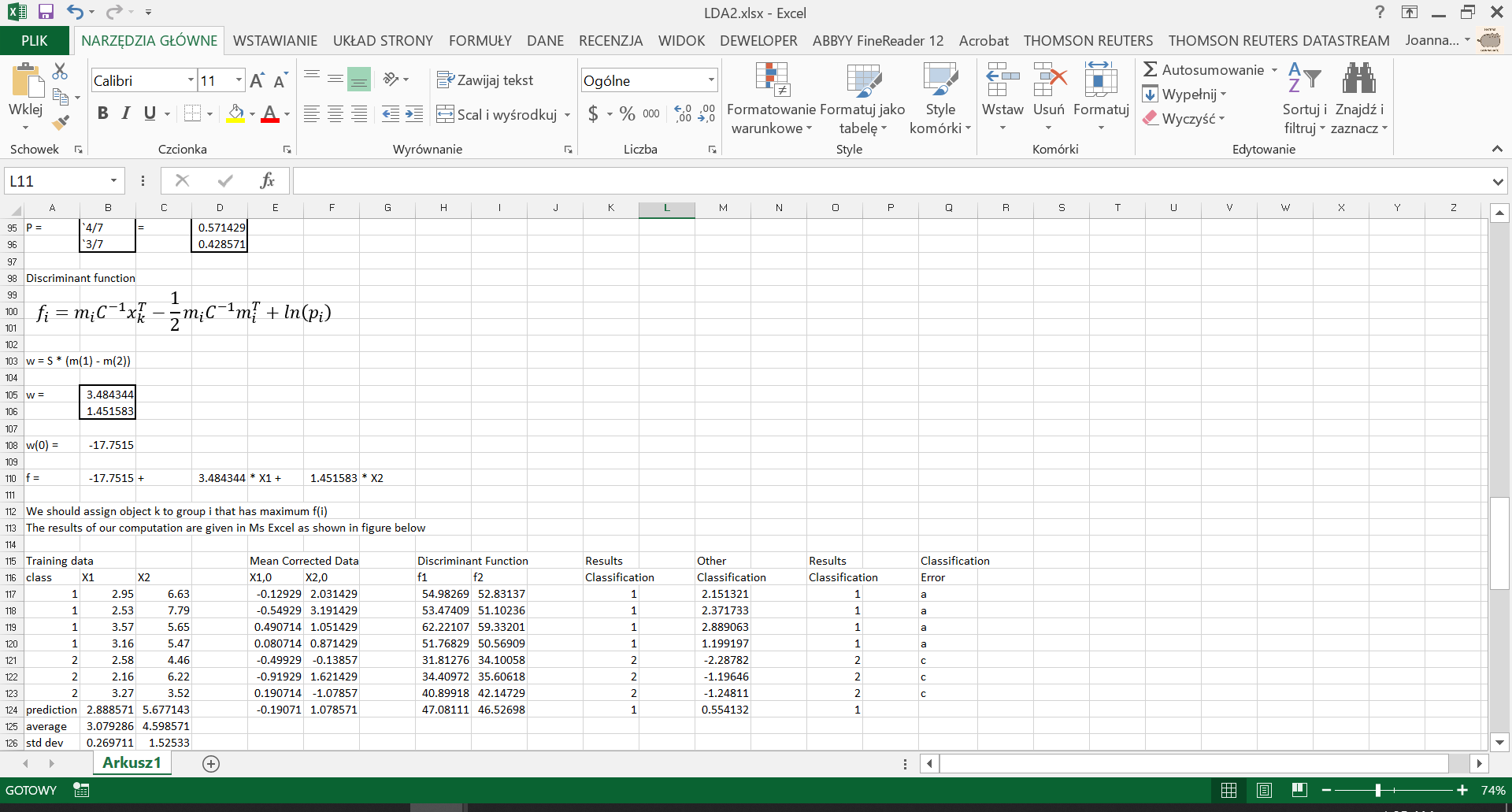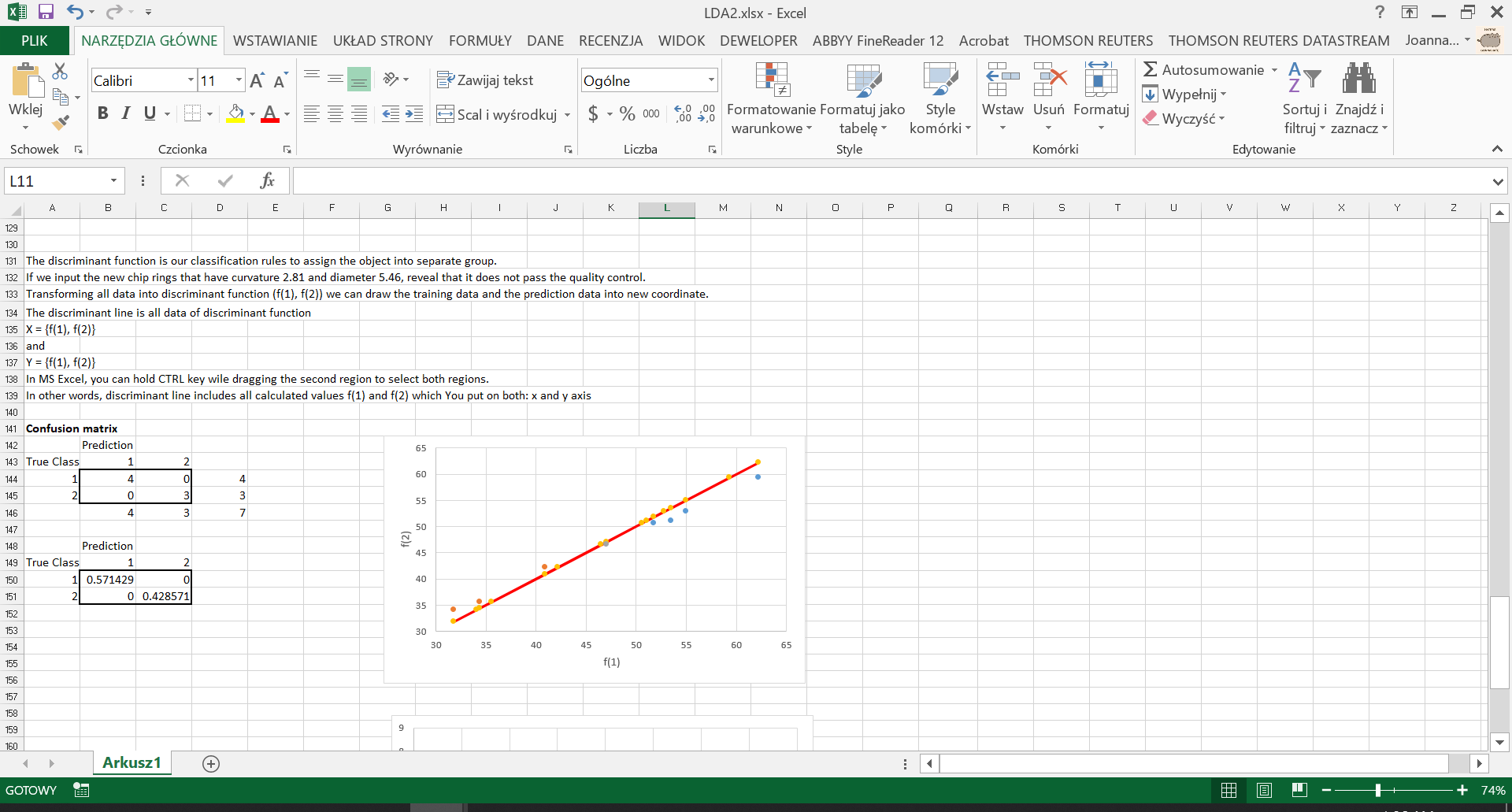Tagged: